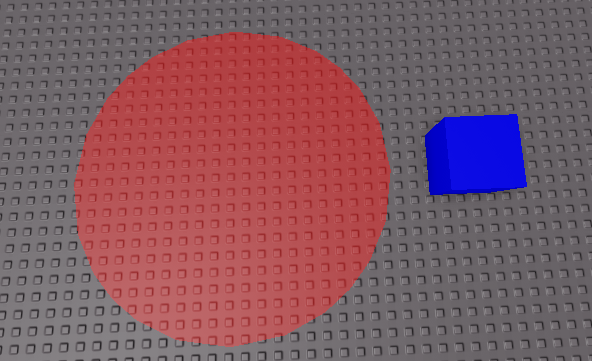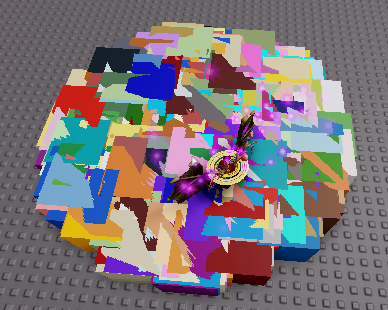# Block positioned in a random place in a specific area

I’m working on a project that needs a part to be positioned in a random place (inside the red part)
but I have no idea how to do this, but I need a lot …

here is the red part (Part where the blue block should be positioned randomly within the red area)Since you need a random position inside a circle you could use polar coordinates.

``````local center = -- circle center

local randomAngle = math.random() * math.pi * 2
-- rotate by Angle then move out by Radius
local position = (CFrame.new(center) * CFrame.Angles(0, randomAngle, 0) * CFrame.new(randomRadius, 0, 0)).Position

block.Position = position
``````

this pointing this errorhttp://mathworld.wolfram.com/DiskPointPicking.html
This will suffer from having a distribution like one on the left.

``````local center = -- circle center

local randomAngle = math.random() * math.pi * 2
-- rotate by Angle then move out by Radius
local position = (CFrame.new(center) * CFrame.Angles(0, randomAngle, 0) * CFrame.new(randomRadius, 0, 0)).Position

block.Position = position
``````

I’ve added a math.sqrt() to fix that issue and it now gives a distribution like the one on the right (within that link).

1 Like

Is your script cloning itself somehow causing more instances of the same script to run and clone more copies of itself?

yes,this is my script that i am using to test if it will work correctly in my game

``````local Circleposition = script.Parent.Position

local randomAngle = math.random() * math.pi * 2

script.Parent.Transparency = 1

for i = 1,5 do

local Clone = script.Parent:Clone()

Clone.BrickColor = BrickColor.Random()

Clone.Transparency = 0

local position = (CFrame.new(Circleposition) * CFrame.Angles(0, randomAngle, 0) * CFrame.new(randomRadius, 0, 0)).Position

Clone.Position = position

Clone.Parent = workspace

end
``````

and for some reason it is cloning well more than just 5 timesThis clones the parent of the script. The new clone also contains a clone of the same script. Hence, the script is creating more and more copies of itself. You want to avoid cloning the script over when you clone your script.Parent. You could add a Clone:ClearAllChildren() underneath that line to make sure the clone doesn’t contain a copy of the same script.

Also, since you are picking the random coordinates outside the loop, you are assigning the same position to all 5 of the cloned blocks.

it’s working, thanks to those who helped me